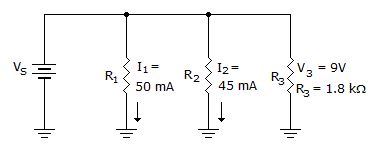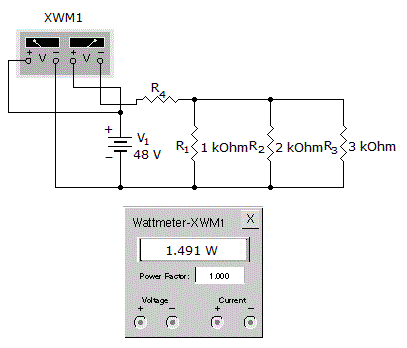# Electronics - Parallel Circuits

### Exercise :: Parallel Circuits - General Questions

36.What does R1 equal in the given circuit?

 A. 90B. 180C. 200D. 1.8 kExplanation:

No answer description available for this question. Let us discuss.

37.

What is the total resistance?A. 1.045 kB. 1.545 kC. 2.045 kD. 2.545 kExplanation:

No answer description available for this question. Let us discuss.

38.

What is RT for fifteen 2 Mresistors all connected in parallel?

 A. 133 kB. 300 kC. 750 kD. 30 MExplanation:

No answer description available for this question. Let us discuss.

39.

Which is considered to be the common reference for a parallel circuit?

 A. Voltage B. Current C. Power D. Resistance